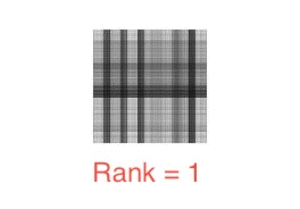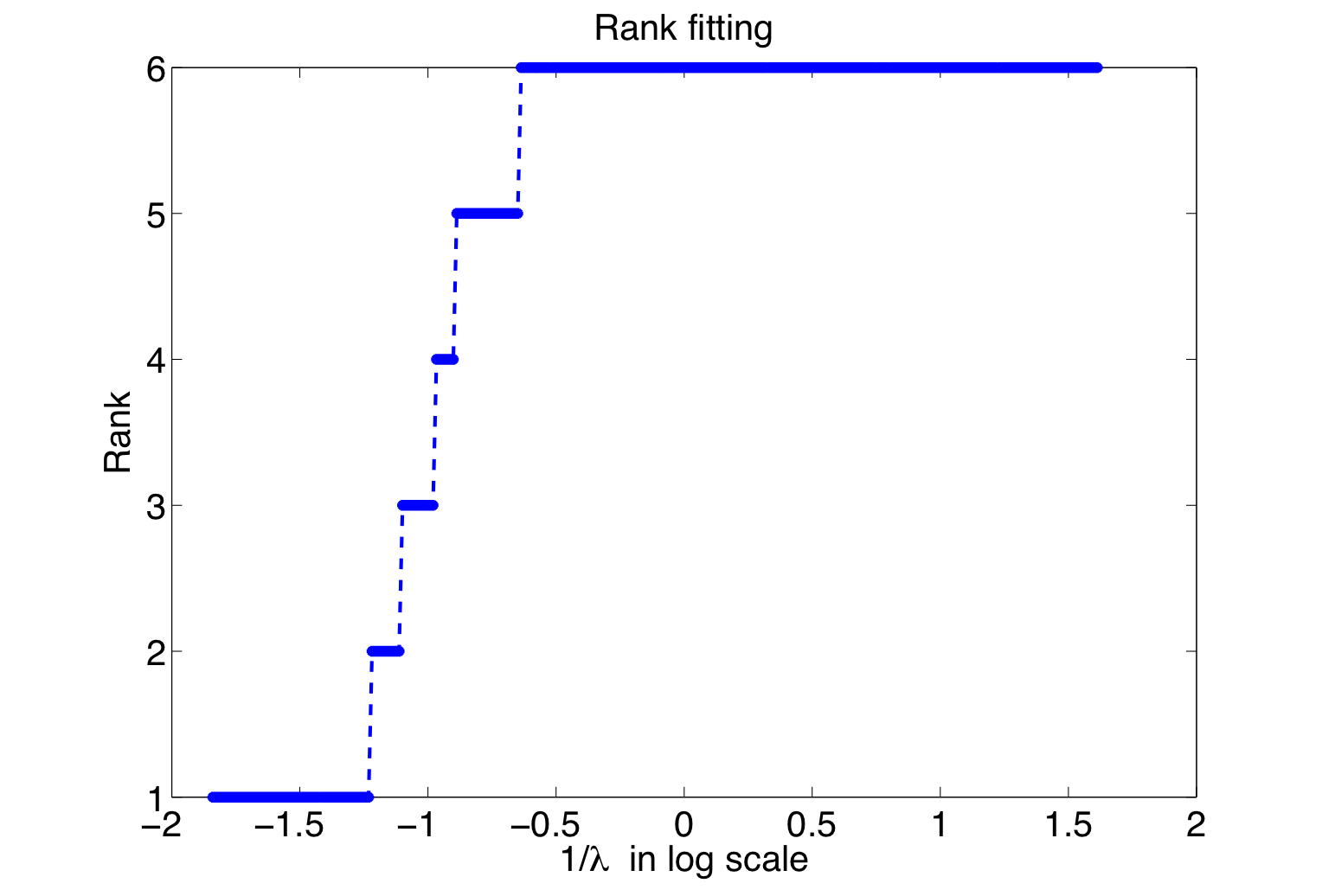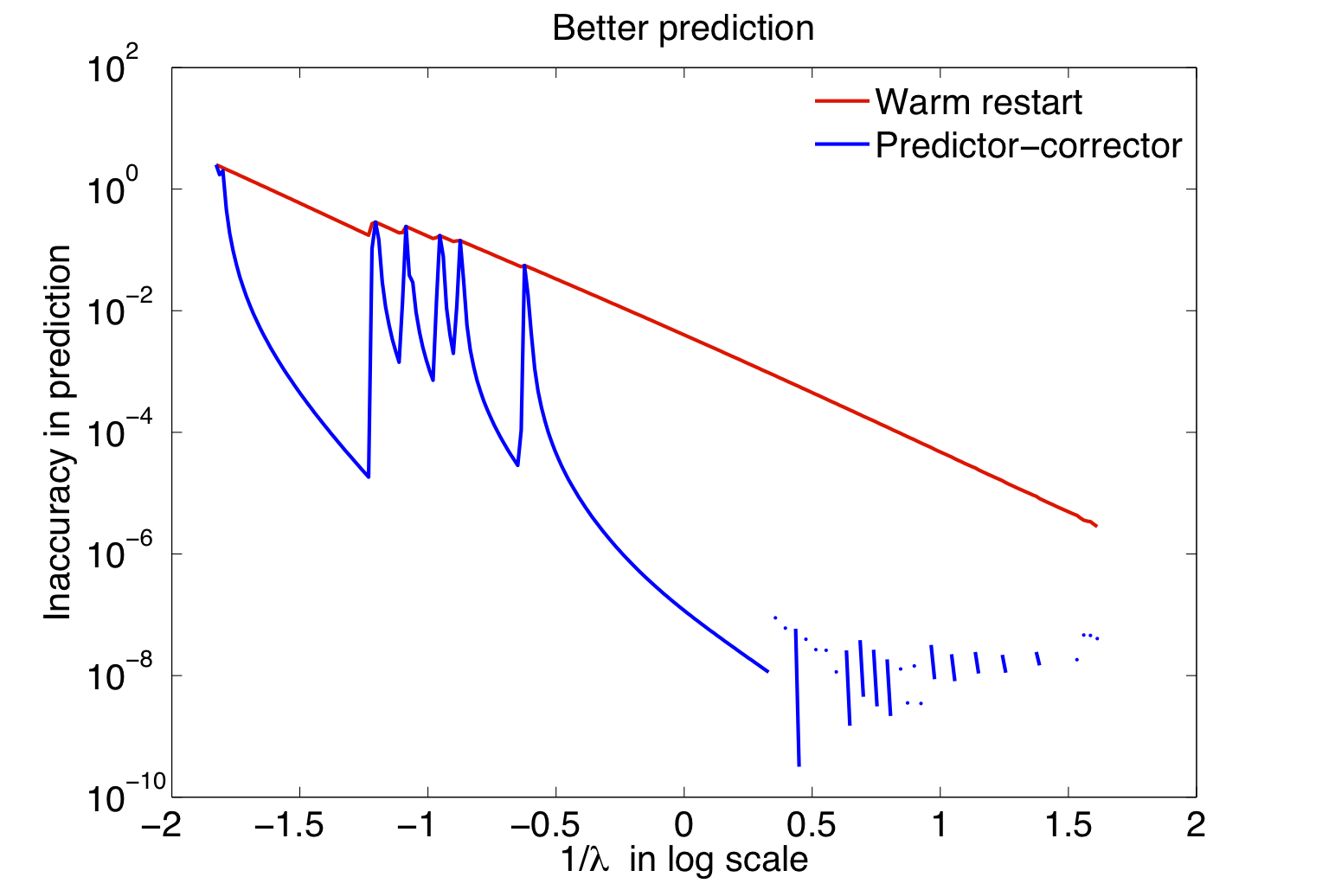## Bamdev Mishra

#### Authors

B. Mishra, G. Meyer, F. Bach, and R. Sepulchre

#### Abstract

Problems of the form f(X) + λ||X||* are tackled, where f is a smooth convex function, ||X||* is the trace (or nuclear) norm of X, and λ >= 0 is a regularization parameter.

The paper addresses the problem of low-rank trace norm (also known as nuclear norm) minimization. We propose an algorithm that alternates between fixed-rank optimization and rank-one updates. The fixed-rank optimization is characterized by an efficient factorization that makes the trace norm differentiable in the search space and the computation of duality gap numerically tractable. The search space is nonlinear but is equipped with a particular Riemannian structure that leads to efficient computations. We present a second-order trust-region algorithm with a guaranteed quadratic rate of convergence. Overall, the proposed optimization scheme converges super-linearly to the global solution while still maintaining complexity that is linear in the number of rows of the matrix. To compute a set of solutions efficiently for a grid of regularization parameters we propose a predictor-corrector approach on the quotient manifold that outperforms the naive warm-restart approach. The performance of the proposed algorithm is illustrated on problems of low-rank matrix completion and multivariate linear regression.Recovered image with different ranksTraversing different ranks.Original imageBetter prediction accuracy of regularization path